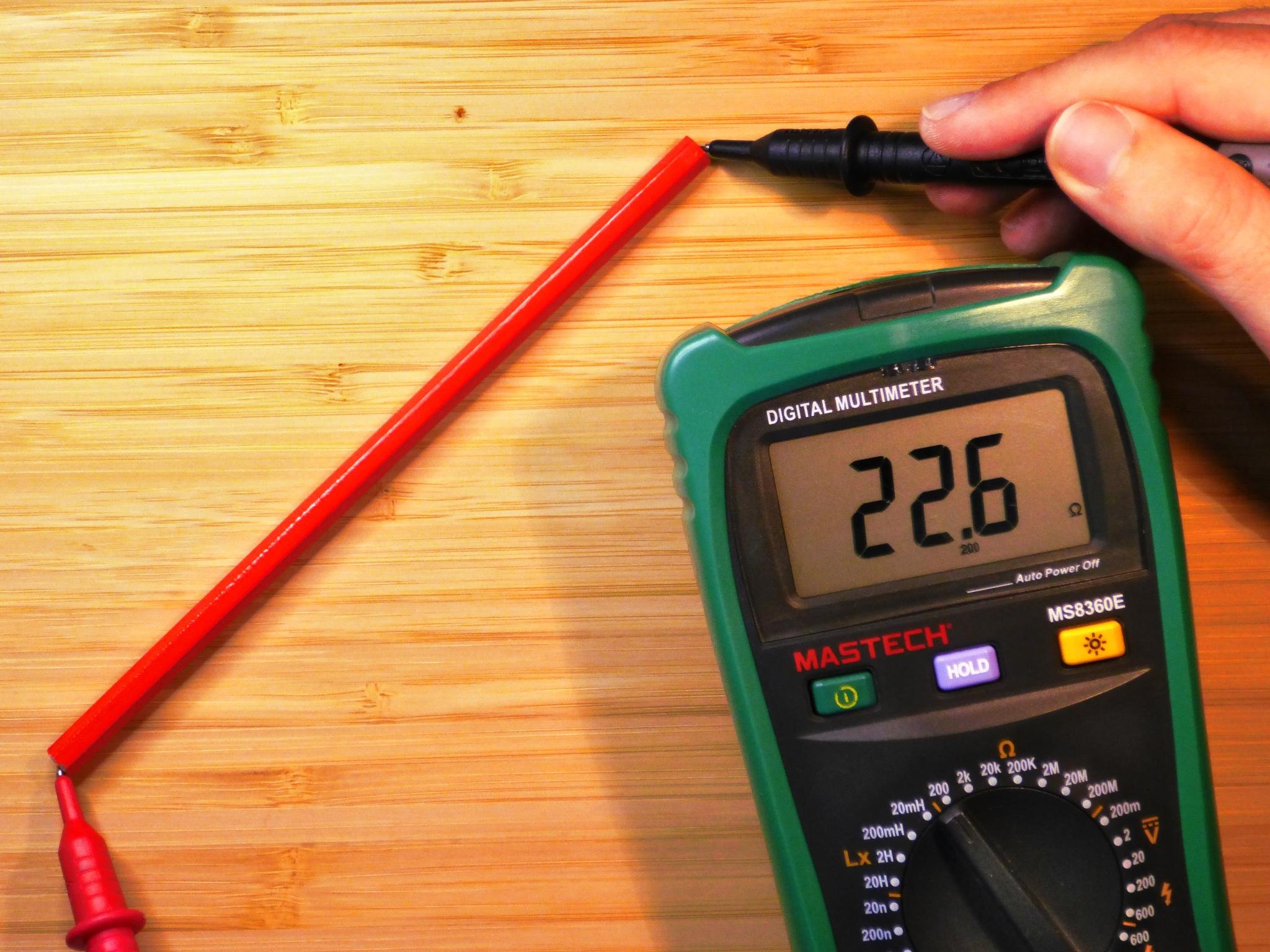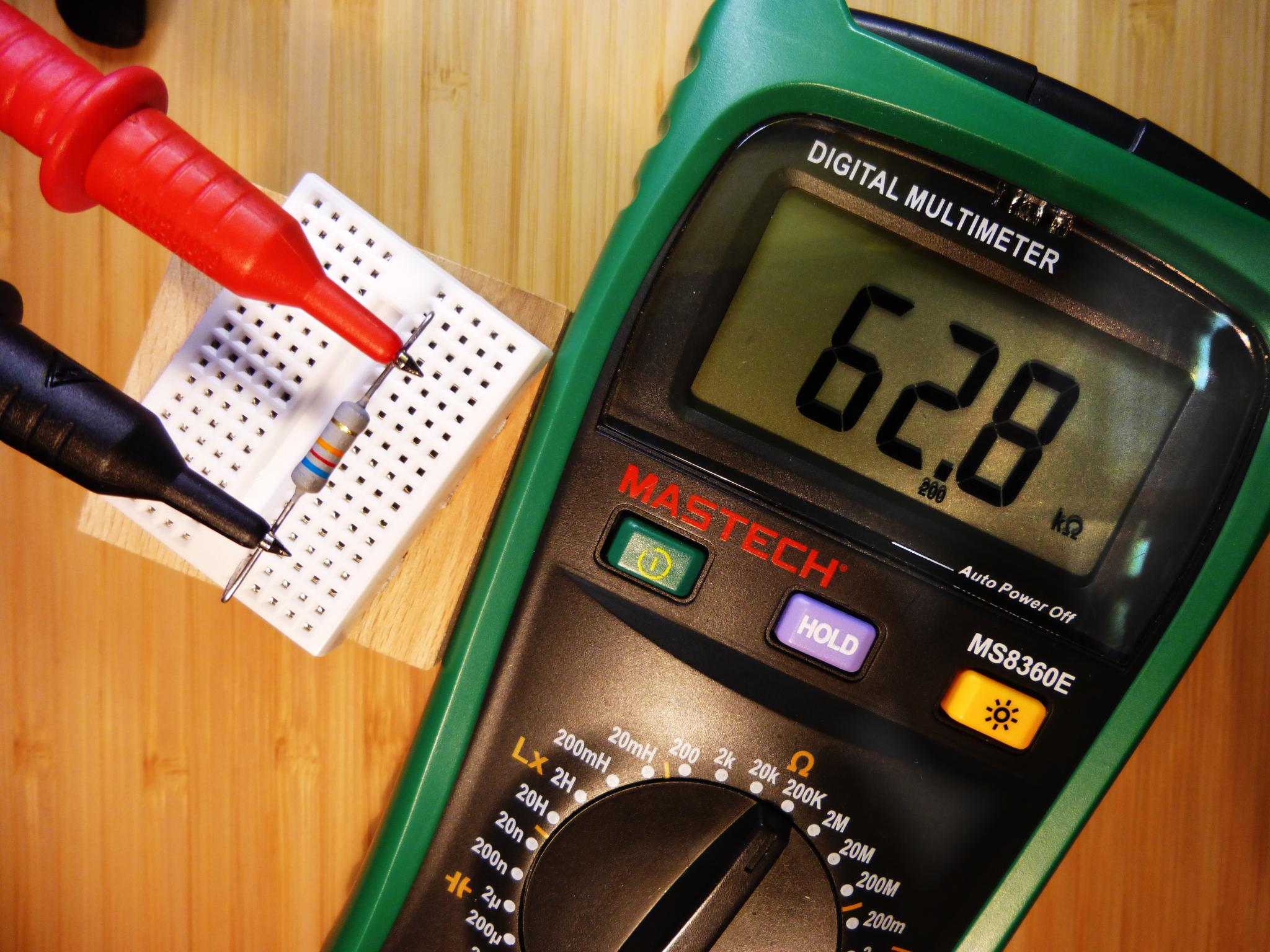# Conductivity and Resistance

Among the components found in circuits, resistors are pretty common. Let's take a look at them and the conductivity of different materials.

## Resistivity and Conductivity

Conductivity is the ability of a material to transport charges, either electrons or ions. This differs from material to material. It is however, not common for materials to allow for free electron flow. In most materials, electrons are either captured in chemical bindings, or to close to the atom core to be separated from it.

Especially metals are good conductors. The conductivity does not solely depend on the chemical elements in the material but also on its structure. Diamonds which consist out of carbon does not conduct, while graphite, also carbon, does.

Metals form a grid of atoms in which the outer electrons are very loosely coupled to the atom core. This is why they can be easily disassociated form it and move inside the material. Metals conduct. The situation in graphite is quite similar. Graphite consist of grids of carbon atoms layered over each other. The binding between these layers is pretty weak and the electrons that take part in it can be disassociated from their atom cores as well. The layered structure in graphite allows us to use it in pencils. When drawing on a piece of paper, the graphite lead is rubbed off layer by layer. The layered structure has strange effect on the conductivity too. Only in between the layers are moveable electrons. For this reason graphite conducts better if the current passes parallel to the layers, but a lot worse if we try to force the electrons to move perpendicular to the graphite layers.

We covered most of the conductive materials with this selection. There are not a lot of conductive materials around us. Most materials are pretty bad conductors. If they conduct badly enough, they can be used as isolators. Semiconductors like silicon naturally has a conductivity that lays in between of the conductivity of metals and other badly conductive materials. This is the reason for their name. Today, we know that they have a really special behavior we can make use of in transistors and other electronic components. But I won't cover this now, as this is a pretty complex topic for itself.

Another interesting topic, which we will cover now, is the conductivity of water. Water is conductive because there are ions in it which can freely move and transport charges. Normal tap water is not a good conductor, but it is conductive enough that we could get killed in case of a lightning strike while swimming. If we add more ions, for instance by adding salt we can greatly improve its conductivity. This is experiment is shown in the picture below.

Conductivity ($$\sigma$$) is measure in the unit Siemens per meter ($$S \over m$$).
You probably are more familiar with the inverse of conductivity - resistivity ($$\rho$$), resistance ($$R$$) and the unit ohm (Ω).
$$1 S = 1 {1 \over Ω}$$

Conductivity can be easily converted to resistivity using this simple formula: $$\rho = {1 \over \sigma}$$

Resistivity is, however, not commonly given in $$Ωm$$ but in the SI-Unit $${{Ω \cdot {{mm}^2}} \over m}$$ as this is more practical for calculations.
$$1 Ωm = 1 \cdot {10}^6 {{Ω \cdot {{mm}^2}} \over m}$$

Here is a table of resistivity values for some selected materials. The values can hugely differ depending on the source. This is because small changes in material purity and structure can have a huge impact on the measured resistivity value. My values are taken from the English Wikipedia.

Material Conductivity ($$S$$) Resistivity ($$Ωm$$) Resistivity ($${Ω \cdot mm²} \over m$$)
Silver $$6.30 \cdot 10^7$$ $$1.59 \cdot 10 ^ {-8}$$ $$1.59 \cdot 10 ^ {-2}$$
Copper $$5.96 \cdot 10^7$$ $$1.68 \cdot 10 ^ {-8}$$ $$1.68 \cdot 10 ^ {-2}$$
Gold $$4.11 \cdot 10^7$$ $$2.44 \cdot 10^{-8}$$ $$2.44 \cdot 10^{-2}$$
Iron $$10^7$$ $$9.7 \cdot 10^{-8}$$ $$9.7 \cdot 10^{-2}$$
Graphite (parallel) $$2 \cdot 10^5$$ to $$3 \cdot 10^5$$ $$2.5 \cdot 10 ^ {-6}$$ to $$5 \cdot 10^{-6}$$ $$2.5$$ to $$5$$
Graphite (amorphous) $$1.25 \cdot 10^3$$ to $$2.00 \cdot 10^3$$ $$5 \cdot 10^{-4}$$ to $$8 \cdot 10^{-4}$$ $$5 \cdot 10^2$$ to $$8 \cdot 10^2$$
Graphite (perpendicular) $$3.3 \cdot 10^2$$ $$3 \cdot 10^{-3}$$ $$3 \cdot 10^3$$
Sea Water $$4.8$$ $$2 \cdot 10^{-1}$$ $$2 \cdot 10^5$$
Drinking Water $$5 \cdot 10^{−4}$$ to $$5 \cdot 10^{-2}$$ $$2 \cdot 10^1$$ to $$2 \cdot 10^3$$ $$2 \cdot 10^7$$ to $$2 \cdot 10^9$$
Silicon $$4.35 \cdot 10^{−4}$$ $$2.3 \cdot 10^3$$ $$2.3 \cdot 10^{10}$$
Deionized Water $$5.5 \cdot 10^{-6}$$ $$1.8 \cdot 10^5$$ $$1.8 \cdot 10^{12}$$
Glas $$10^{-15}$$ to $$10^{-11}$$ $$10^{11}$$ to $$10^{15}$$ $$10^{17}$$ to $$10^{21}$$
Air $$10^{-15}$$ to $$10^{-9}$$ $$10^9$$ to $$10^{15}$$ $$10^{15}$$ to $$10^{21}$$
PET $$10^{-21}$$ $$10^{21}$$ $$10^{27}$$

# From Resistivity to Resistance

If we have the material specific resistivity, we can use it to calculate the resistance of an object. Resistance is measured in ohm (Ω). The SI-unit of the resistivity already tells us the required formula:
$$R = {{\rho \cdot l} \over A}$$

The resistance $$R$$ of a wire increases with the length $$l$$. On the other hand the resistance decreases with an increasing cross-section $$A$$ of the wire. This is why we have to use thick wires for long distances and high currents.

Let's look at an example were we try out this formula. We won't be able to do this with metal, as we would need really long and thin wires to get a resistance high enough to measure it with our multimeter. There is a more practical example: We will use the graphite mine of a pencil.

My pencil is 17,5 cm long and the lead is 2 mm thick. There is one problem however, we don't know the actual material composition of the lead. It's most likely not pure graphite and also not a fully ordered crystalline structure. This is a general problem when calculating the resistance in practice. We often don't know the internal structure and the purity of the materials we use. Pencil leads are made out of a mixture of graphite powder and clay. This also allows the creation of pencil leads with different grading.

For the graphite powder the resistivity of amorphous graphite should roughly match. Amorphous means that there is no clear crystalline structure, but only small scattered areas of crystalline structures. These crystalline areas have all different orientations. As a consequence there is no direction in which the material will conduct better than the other. As the graphite in our pencil lead was powdered, it is not possible that there is a complete crystalline structure throughout the lead.

Let's do the math:
$$l = 0.175 m$$
$$d = 2 mm$$
$$A = (d/2)^2 \cdot \pi = 1 {mm}^2 \cdot \pi = 3.14 {mm}^2$$

For the lower bound resistivity given:
$$\rho = {5 \cdot 10^2 {Ω \cdot {{mm}^2} \over m}}$$
$$R = {{\rho \cdot l} \over A} = {{{5 \cdot 10^2 {Ω \cdot {{mm}^2} \over m}} \cdot {0.175 m}} \over {3.14 {mm}^2}} \approx 28 Ω$$

For the upper bound resistivity given:
$$\rho = {8 \cdot 10^2 {Ω \cdot {{mm}^2} \over m}}$$
$$R = {{\rho \cdot l} \over A} = {{{8 \cdot 10^2 {Ω \cdot {{mm}^2} \over m}} \cdot {0.175 m}} \over {3.14 {mm}^2}} \approx 45 Ω$$

The resistance should thus be roughly in between 28 Ω and 45 Ω. Of course, we have to expect some deviations as we worked quite imprecise. This starts with the not fully known unknown material composition and ends with the fact that I will not care about the temperature when measuring the real resistance value. Nevertheless, our result is not too bad. I measured 22.6 Ω which is not in our theoretically calculated range for amorphous graphite, but not too far off either. Given our imprecise work process the difference could have been worse.## Resistors

In electronics, we make use of the fact that not all materials are perfect conductors. We can use materials with a higher resistance to artificially limit the current flow in our circuit. This is what resistors are for. They are specifically constructed to have a specific resistance. We could measure their resistance value with a multimeter, but it is typically also printed on the outer shell, sometimes directly but most often encoded with a color code. Here is how this color code works:As you can see there are resistors with a different number of bands. Metal film resistor usually have one additional digit ring. Occasionally you can also find resistors with 6 bands. The additional 6th band specifies how the resistance changes with the temperature. This is only important if you really need a high precision resistor. It is actually quite easy to use this code table. You determine the digits for the first two or three rings and then multiply the number with the multiplier.

Let's take a look at an example: The resistor shown in the picture below has 4 bands with the colors blue, red, orange and gold. We first determine the 2 digits, 6 and 2 and then multiply by 1K. This results in a resistance of 62 kΩ. You can see that the multimeter shows 62.9 kΩ. This is still inside the specs as the resistor has a tolerance of 5% (gold band). It's not hard at all, but it needs some practice. After some time you know most of the colors by heart. There is one caveat however, its sometimes not that easy to tell on which side the first ring is located. Some resistors have a bigger gap between the multiplier and the tolerance ring and if you have a golden tolerance ring it is clear that this is not the first digit, but there are cases in which it is impossible to tell. In these cases I recommend the use of a multimeter to make sure you picked the right resistor.When searching for a resistor with a specific value you might not be able to find it. There is a collection of standard values. For resistors with a tolerance 5% this collection is called E24. The other values are still within the tolerance of next or previous standard value. As a result it is not strictly necessary to have them. If you really need a more accurate value you are advised to use resistors with a smaller tolerance. For resistors with 2% tolerance there is the E48 series includes more resistor values.

We now know about the resistance value of resistors. In circuits with higher currents there is an additional point to keep in mind. The electrons interact with the resistive material while flowing through it. The resistor heats up. If the current is too high the resistor will just burn up. You can calculate the amount of energy that is converted into heat every second by using the following formula:
$$P_{loss} = I^2 \cdot R$$

The small standard resistors usually have a power rating of 0.25 W. We will talk on how resistance, voltage and current behave relative to each other in the next tutorial about ohms law. You will then understand that it is easy to exceed this power rating, especially for small resistance values. An example were this can happen is the use of high power LEDs. It's important to keep that in mind when designing your own circuits.